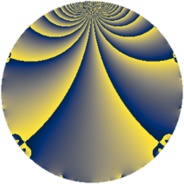# Properties

 Label 2023.2.aLevel $2023$ Weight $2$ Character orbit 2023.a Rep. character $\chi_{2023}(1,\cdot)$ Character field $\Q$ Dimension $135$ Newform subspaces $18$ Sturm bound $408$ Trace bound $6$

# Related objects

## Defining parameters

 Level: $$N$$ $$=$$ $$2023 = 7 \cdot 17^{2}$$ Weight: $$k$$ $$=$$ $$2$$ Character orbit: $$[\chi]$$ $$=$$ 2023.a (trivial) Character field: $$\Q$$ Newform subspaces: $$18$$ Sturm bound: $$408$$ Trace bound: $$6$$ Distinguishing $$T_p$$: $$2$$, $$3$$

## Dimensions

The following table gives the dimensions of various subspaces of $$M_{2}(\Gamma_0(2023))$$.

Total New Old
Modular forms 222 135 87
Cusp forms 187 135 52
Eisenstein series 35 0 35

The following table gives the dimensions of the cuspidal new subspaces with specified eigenvalues for the Atkin-Lehner operators and the Fricke involution.

$$7$$$$17$$FrickeDim.
$$+$$$$+$$$$+$$$$31$$
$$+$$$$-$$$$-$$$$36$$
$$-$$$$+$$$$-$$$$40$$
$$-$$$$-$$$$+$$$$28$$
Plus space$$+$$$$59$$
Minus space$$-$$$$76$$

## Trace form

 $$135 q - q^{2} + 131 q^{4} - 2 q^{5} + q^{7} + 3 q^{8} + 127 q^{9} + O(q^{10})$$ $$135 q - q^{2} + 131 q^{4} - 2 q^{5} + q^{7} + 3 q^{8} + 127 q^{9} - 2 q^{10} + 16 q^{12} - 14 q^{13} + 3 q^{14} + 4 q^{15} + 135 q^{16} + 19 q^{18} - 20 q^{19} + 22 q^{20} - 4 q^{21} + 16 q^{22} + 16 q^{23} + 24 q^{24} + 121 q^{25} + 30 q^{26} + 24 q^{27} + 7 q^{28} + 6 q^{29} + 36 q^{30} - 12 q^{31} - 5 q^{32} - 8 q^{33} - 2 q^{35} + 115 q^{36} - 14 q^{37} + 8 q^{38} + 4 q^{39} - 22 q^{40} - 30 q^{41} - 12 q^{42} - 8 q^{44} + 2 q^{45} - 32 q^{46} + 8 q^{47} + 12 q^{48} + 135 q^{49} - 35 q^{50} - 46 q^{52} + 22 q^{53} - 28 q^{54} + 15 q^{56} - 36 q^{57} + 10 q^{58} - 4 q^{59} - 36 q^{60} - 34 q^{61} - 32 q^{62} + 5 q^{63} + 143 q^{64} + 4 q^{65} - 48 q^{66} - 20 q^{67} + 16 q^{69} + 6 q^{70} + 16 q^{71} - 25 q^{72} - 30 q^{73} + 22 q^{74} + 44 q^{75} - 48 q^{76} - 4 q^{77} - 12 q^{78} - 4 q^{79} + 14 q^{80} + 103 q^{81} + 14 q^{82} + 32 q^{83} - 28 q^{84} - 68 q^{86} + 4 q^{87} + 68 q^{88} - 18 q^{89} - 70 q^{90} - 6 q^{91} - 32 q^{92} + 40 q^{93} + 12 q^{94} + 36 q^{95} - 38 q^{97} - q^{98} + 60 q^{99} + O(q^{100})$$

## Decomposition of $$S_{2}^{\mathrm{new}}(\Gamma_0(2023))$$ into newform subspaces

Label Dim. $$A$$ Field CM Traces A-L signs $q$-expansion
$$a_2$$ $$a_3$$ $$a_5$$ $$a_7$$ 7 17
2023.2.a.a $$1$$ $$16.154$$ $$\Q$$ None $$-1$$ $$-3$$ $$-4$$ $$1$$ $$-$$ $$+$$ $$q-q^{2}-3q^{3}-q^{4}-4q^{5}+3q^{6}+q^{7}+\cdots$$
2023.2.a.b $$1$$ $$16.154$$ $$\Q$$ None $$-1$$ $$3$$ $$4$$ $$-1$$ $$+$$ $$-$$ $$q-q^{2}+3q^{3}-q^{4}+4q^{5}-3q^{6}-q^{7}+\cdots$$
2023.2.a.c $$3$$ $$16.154$$ 3.3.148.1 None $$-1$$ $$-1$$ $$-2$$ $$-3$$ $$+$$ $$+$$ $$q-\beta _{1}q^{2}-\beta _{1}q^{3}+(1+\beta _{1}+\beta _{2})q^{4}+\cdots$$
2023.2.a.d $$3$$ $$16.154$$ 3.3.148.1 None $$-1$$ $$1$$ $$2$$ $$3$$ $$-$$ $$-$$ $$q-\beta _{1}q^{2}+\beta _{1}q^{3}+(1+\beta _{1}+\beta _{2})q^{4}+\cdots$$
2023.2.a.e $$4$$ $$16.154$$ 4.4.9301.1 None $$-1$$ $$-2$$ $$-2$$ $$-4$$ $$+$$ $$+$$ $$q-\beta _{1}q^{2}+(-1-\beta _{3})q^{3}+(1+\beta _{1}+\beta _{2}+\cdots)q^{4}+\cdots$$
2023.2.a.f $$4$$ $$16.154$$ 4.4.2225.1 None $$3$$ $$-2$$ $$-4$$ $$-4$$ $$+$$ $$-$$ $$q+(1-\beta _{1})q^{2}+\beta _{2}q^{3}+(2-\beta _{1}+\beta _{2}+\cdots)q^{4}+\cdots$$
2023.2.a.g $$4$$ $$16.154$$ 4.4.2225.1 None $$3$$ $$2$$ $$4$$ $$4$$ $$-$$ $$+$$ $$q+(1-\beta _{1})q^{2}-\beta _{2}q^{3}+(2-\beta _{1}+\beta _{2}+\cdots)q^{4}+\cdots$$
2023.2.a.h $$5$$ $$16.154$$ 5.5.240133.1 None $$2$$ $$0$$ $$-4$$ $$-5$$ $$+$$ $$+$$ $$q+\beta _{1}q^{2}+(-\beta _{1}+\beta _{4})q^{3}+(\beta _{1}+\beta _{2}+\cdots)q^{4}+\cdots$$
2023.2.a.i $$5$$ $$16.154$$ 5.5.240133.1 None $$2$$ $$0$$ $$4$$ $$5$$ $$-$$ $$+$$ $$q+\beta _{1}q^{2}+(\beta _{1}-\beta _{4})q^{3}+(\beta _{1}+\beta _{2})q^{4}+\cdots$$
2023.2.a.j $$5$$ $$16.154$$ 5.5.453749.1 None $$2$$ $$2$$ $$0$$ $$5$$ $$-$$ $$+$$ $$q+\beta _{4}q^{2}+(\beta _{1}-\beta _{3})q^{3}+(2-\beta _{3})q^{4}+\cdots$$
2023.2.a.k $$9$$ $$16.154$$ 9.9.$$\cdots$$.1 None $$-6$$ $$0$$ $$0$$ $$9$$ $$-$$ $$-$$ $$q+(-1-\beta _{5})q^{2}-\beta _{4}q^{3}+(1-\beta _{3}+\beta _{5}+\cdots)q^{4}+\cdots$$
2023.2.a.l $$9$$ $$16.154$$ 9.9.$$\cdots$$.1 None $$-6$$ $$0$$ $$0$$ $$-9$$ $$+$$ $$+$$ $$q+(-1-\beta _{5})q^{2}+\beta _{4}q^{3}+(1-\beta _{3}+\beta _{5}+\cdots)q^{4}+\cdots$$
2023.2.a.m $$10$$ $$16.154$$ $$\mathbb{Q}[x]/(x^{10} - \cdots)$$ None $$0$$ $$-4$$ $$-8$$ $$-10$$ $$+$$ $$+$$ $$q+\beta _{1}q^{2}-\beta _{8}q^{3}+(2+\beta _{7}-\beta _{8})q^{4}+\cdots$$
2023.2.a.n $$10$$ $$16.154$$ $$\mathbb{Q}[x]/(x^{10} - \cdots)$$ None $$0$$ $$4$$ $$8$$ $$10$$ $$-$$ $$+$$ $$q+\beta _{1}q^{2}+\beta _{8}q^{3}+(2+\beta _{7}-\beta _{8})q^{4}+\cdots$$
2023.2.a.o $$15$$ $$16.154$$ $$\mathbb{Q}[x]/(x^{15} - \cdots)$$ None $$6$$ $$0$$ $$0$$ $$15$$ $$-$$ $$+$$ $$q+\beta _{1}q^{2}+(-\beta _{1}+\beta _{13})q^{3}+(1+\beta _{2}+\cdots)q^{4}+\cdots$$
2023.2.a.p $$15$$ $$16.154$$ $$\mathbb{Q}[x]/(x^{15} - \cdots)$$ None $$6$$ $$0$$ $$0$$ $$-15$$ $$+$$ $$-$$ $$q+\beta _{1}q^{2}+(\beta _{1}-\beta _{13})q^{3}+(1+\beta _{2}+\beta _{4}+\cdots)q^{4}+\cdots$$
2023.2.a.q $$16$$ $$16.154$$ $$\mathbb{Q}[x]/(x^{16} - \cdots)$$ None $$-4$$ $$-8$$ $$-16$$ $$16$$ $$-$$ $$-$$ $$q-\beta _{1}q^{2}+(-1-\beta _{4})q^{3}+(1+\beta _{2})q^{4}+\cdots$$
2023.2.a.r $$16$$ $$16.154$$ $$\mathbb{Q}[x]/(x^{16} - \cdots)$$ None $$-4$$ $$8$$ $$16$$ $$-16$$ $$+$$ $$-$$ $$q-\beta _{1}q^{2}+(1+\beta _{4})q^{3}+(1+\beta _{2})q^{4}+\cdots$$

## Decomposition of $$S_{2}^{\mathrm{old}}(\Gamma_0(2023))$$ into lower level spaces

$$S_{2}^{\mathrm{old}}(\Gamma_0(2023)) \cong$$ $$S_{2}^{\mathrm{new}}(\Gamma_0(17))$$$$^{\oplus 4}$$$$\oplus$$$$S_{2}^{\mathrm{new}}(\Gamma_0(119))$$$$^{\oplus 2}$$$$\oplus$$$$S_{2}^{\mathrm{new}}(\Gamma_0(289))$$$$^{\oplus 2}$$